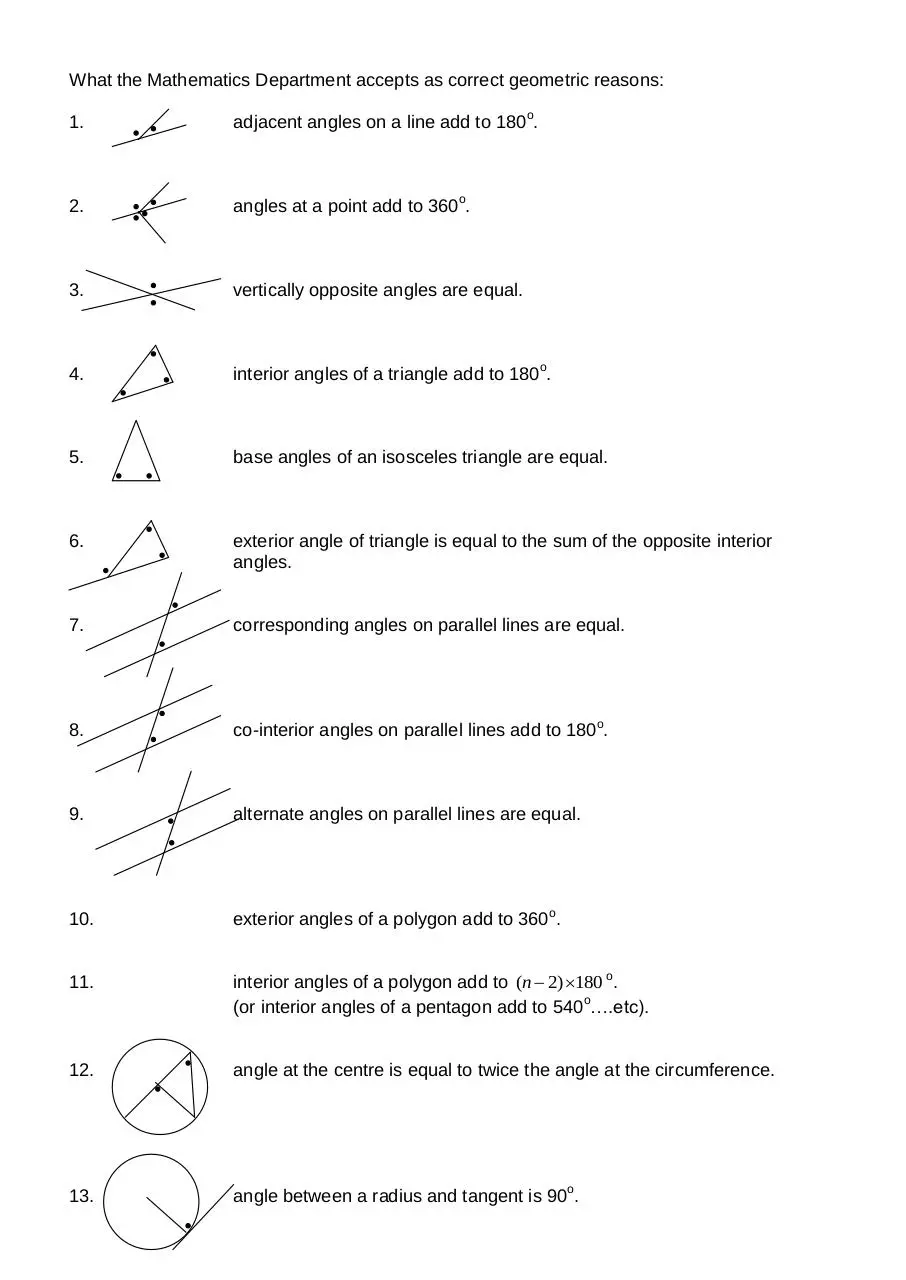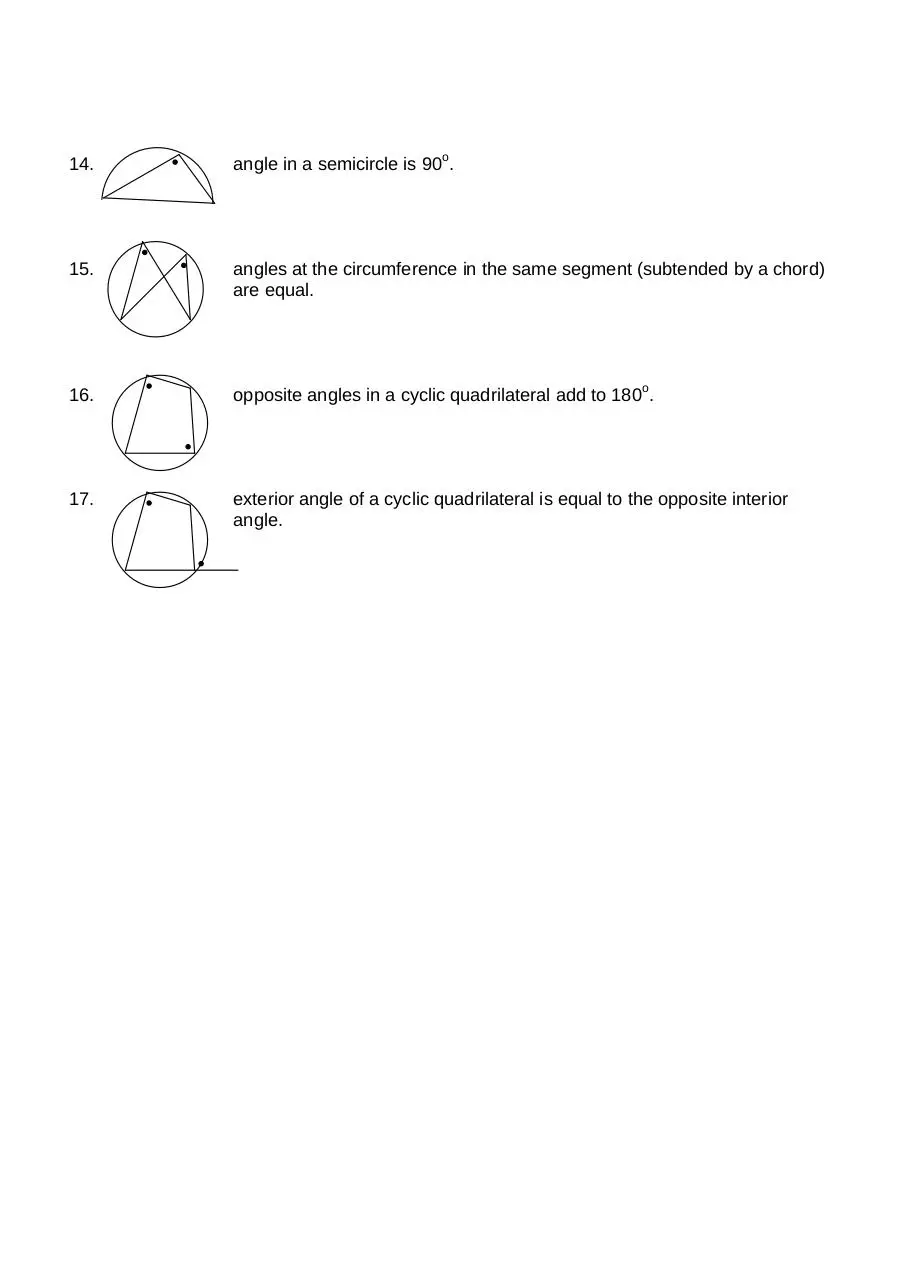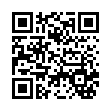# Geometric Rules and Reasons .pdf

### File information

Title: What fussy Ruth would accept as geometric reasons:
Author: Ministry of Education

This PDF 1.5 document has been generated by MicrosoftÂ® Word 2010, and has been sent on pdf-archive.com on 24/06/2013 at 09:53, from IP address 118.92.x.x. The current document download page has been viewed 6190 times.
File size: 82.87 KB (2 pages).
Privacy: public file

### Document preview

What the Mathematics Department accepts as correct geometric reasons:
1.

2.

angles at a point add to 360o.

3.

vertically opposite angles are equal.

4.

interior angles of a triangle add to 180o.

5.

base angles of an isosceles triangle are equal.

6.

exterior angle of triangle is equal to the sum of the opposite interior
angles.

7.

corresponding angles on parallel lines are equal.

8.

co-interior angles on parallel lines add to 180o.

9.

alternate angles on parallel lines are equal.

10.

exterior angles of a polygon add to 360o.

11.

interior angles of a polygon add to (n  2) 180 o.
(or interior angles of a pentagon add to 540o….etc).

12.

angle at the centre is equal to twice the angle at the circumference.

13.

angle between a radius and tangent is 90o.

14.

angle in a semicircle is 90o.

15.

angles at the circumference in the same segment (subtended by a chord)
are equal.

16.

17.

exterior angle of a cyclic quadrilateral is equal to the opposite interior
angle.Geometric_Rules_and_Reasons.pdf (PDF, 82.87 KB)

### Share on social networks

#### HTML Code

Copy the following HTML code to share your document on a Website or Blog